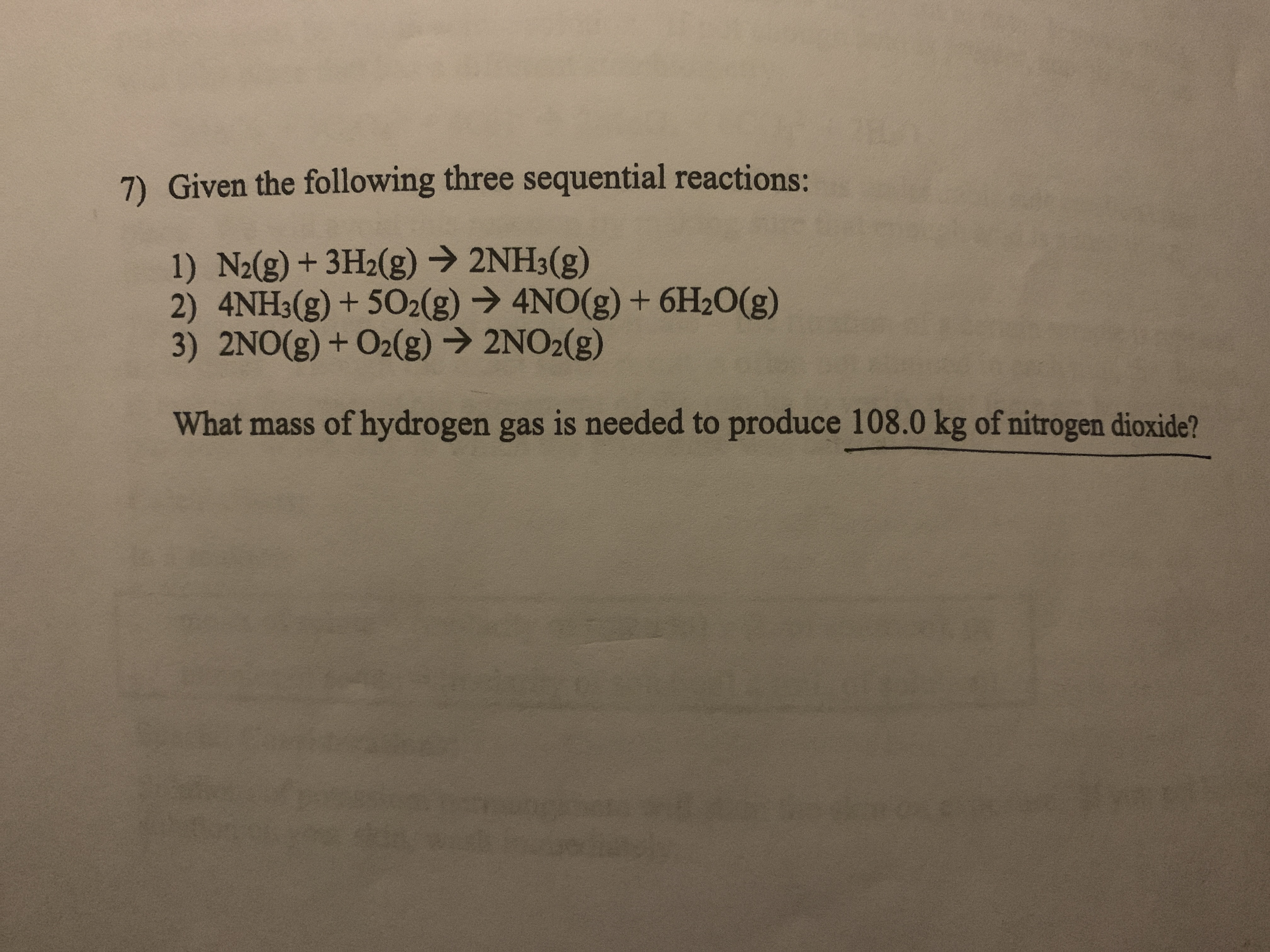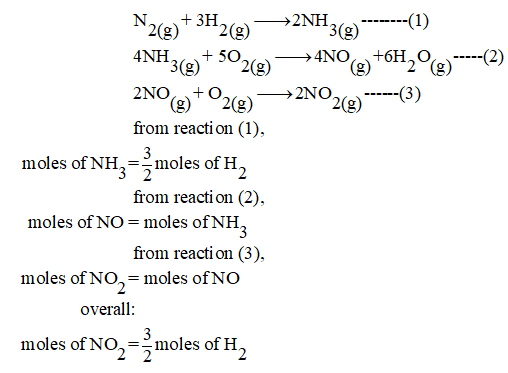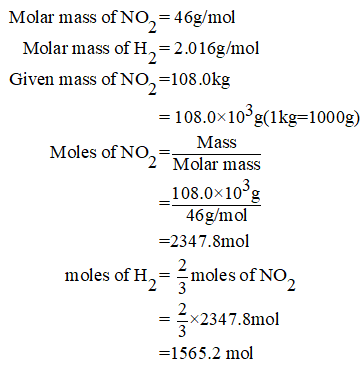# 7) Given the following three sequential reactions:1) N2(g) + 3H2(g) → 2NH3(g)2) 4NH3(g) + 502(g) → 4NO(g) + 6H2O(g)3) 2NO(g) + O2(g)→ 2NO2(g)What mass of hydrogen gas is needed to produce 108.0 kg of nitrogen dioxide?

Question
56 viewshelp_outlineImage Transcriptionclose7) Given the following three sequential reactions: 1) N2(g) + 3H2(g) → 2NH3(g) 2) 4NH3(g) + 502(g) → 4NO(g) + 6H2O(g) 3) 2NO(g) + O2(g)→ 2NO2(g) What mass of hydrogen gas is needed to produce 108.0 kg of nitrogen dioxide? fullscreen
check_circle

Step 1

From the given sequence of reaction;Step 2

The mass of nitrogen dioxide = 108.0kg.  the moles of hydrogen needed to produce the given mass of nitrogen dioxide is calculated as:...

### Want to see the full answer?

See Solution

#### Want to see this answer and more?

Solutions are written by subject experts who are available 24/7. Questions are typically answered within 1 hour.*

See Solution
*Response times may vary by subject and question.
Tagged in